# MONV Documentation

### 0.5

Date:
Oct--Nov 2009
Acknowledgements:
I would like to thank Andrew Lewis from the Computer Laboratory at the University of Cambridge for helpful discussions.

## Overview

The aim of this work is to provide a basic but very thin and fast implementation of monomials with only minimal overhead. The main step in order to achieve this is encoding a given monomial in one machine word, or possibly two, although this case is handled transparently. As a consequence of this design decision, this implementation can only be used for monomials in a fixed small number of variables and with small non-negative exponents.

## Detailed introduction

We assume that a number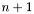of variables is fixed from the beginning, where, and that we only consider monomials with non-negative partial degrees of at most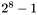. Using 8 bits per exponent, we can encode the monomial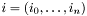as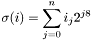in a 64-bit word.

It turns out that, for many operations on monomials, this form is very convenient in the sense that the operation can be performed onas one word instead of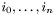involvingwords. Examples of this are multiplication and division, which correspond to addition and subtraction, and the inverse lexicographical order, which corresponds to the operator <. As an aside, the problem of possible overflow is burried in the our assumption that no monomial operation will involve partial exponents exceeding. Other operations, for example, checking whether one monomial is divisible by another, involve explicitly considering partial exponents using bitmasks.

## Monomial orderings

The following (global) monomial orderings are typically considered:

• Lexicographic ordering (lex).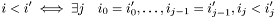• Inverse lexicographic ordering (invlex).• Degree lexicographic (deglex).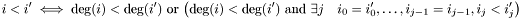• Degree reverse lexicographic (degrevlex).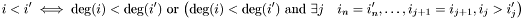In this context, it is very useful to note that, in the inverse lexicographic ordering, we have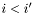if and only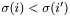. While the first test could involveword comparisons plus the overhead of a loop, the second test only involves one word comparison.

Generated on Sat Nov 14 23:24:59 2009 for MONV by1.5.6